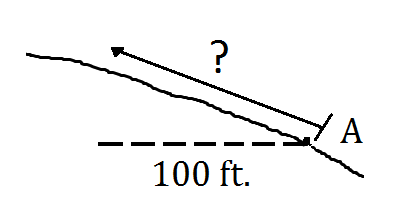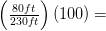# 1.4: Summary Problems

$$\newcommand{\vecs}{\overset { \rightharpoonup} {\mathbf{#1}} }$$ $$\newcommand{\vecd}{\overset{-\!-\!\rightharpoonup}{\vphantom{a}\smash {#1}}}$$$$\newcommand{\id}{\mathrm{id}}$$ $$\newcommand{\Span}{\mathrm{span}}$$ $$\newcommand{\kernel}{\mathrm{null}\,}$$ $$\newcommand{\range}{\mathrm{range}\,}$$ $$\newcommand{\RealPart}{\mathrm{Re}}$$ $$\newcommand{\ImaginaryPart}{\mathrm{Im}}$$ $$\newcommand{\Argument}{\mathrm{Arg}}$$ $$\newcommand{\norm}{\| #1 \|}$$ $$\newcommand{\inner}{\langle #1, #2 \rangle}$$ $$\newcommand{\Span}{\mathrm{span}}$$ $$\newcommand{\id}{\mathrm{id}}$$ $$\newcommand{\Span}{\mathrm{span}}$$ $$\newcommand{\kernel}{\mathrm{null}\,}$$ $$\newcommand{\range}{\mathrm{range}\,}$$ $$\newcommand{\RealPart}{\mathrm{Re}}$$ $$\newcommand{\ImaginaryPart}{\mathrm{Im}}$$ $$\newcommand{\Argument}{\mathrm{Arg}}$$ $$\newcommand{\norm}{\| #1 \|}$$ $$\newcommand{\inner}{\langle #1, #2 \rangle}$$ $$\newcommand{\Span}{\mathrm{span}}$$$$\newcommand{\AA}{\unicode[.8,0]{x212B}}$$

## 1.4 Summary Problems

1. Using the measurements provided, determine the %slope of the following slopes between Points A and B.2. On a 60%slope, Todd wants to walk up a slope a distance equivalent to 100 feet horizontal distance. How far should he walk from Point A?3. On the contour maps below, determine the average slopes between Points A and B. The scale is 1”=2000’. The contour interval is 80’.1.%slope1a.111%

1b.35%

2. On a 60% slope, we know that the rise is 60% of the run. Therefore, the rise here should be 60% of 100 feet or 60 feet. Using the Pythagorean Theorem, we can solve for the hypotenuse.

a2 + b2 = c2 where:

1002 + 602 = c2

13,600 = c2ft.3. The answers to these questions will depend upon how you measured the horizontal, or map distance – hard to do on a screen. My measurements are shown on the maps below:At left. Point A is ≈ 3440’. Point B is ≈ 3720’. The rise is 280’. The run is ≈ 2200’. Therefore, the average slope is (280)(100)/2200 = 13%.

At right. Point A is ≈ 4040’. Point B is ≈ 3280’. The rise is 760’. The run is 1300’. Therefore, the average slope is (760)(100)/2200 = 58%

This page titled 1.4: Summary Problems is shared under a CC BY license and was authored, remixed, and/or curated by Joan DeYoung (OpenOregon) .